# Some Basic Theorems on Trees

Tree:- A connected graph without any circuit is called a Tree. In other words, a tree is an undirected graph G that satisfies any of the following equivalent conditions:

• Any two vertices in G can be connected by a unique simple path.
• G is acyclic, and a simple cycle is formed if any edge is added to G.
• G is connected and has no cycles.
• G is connected but would become disconnected if any single edge is removed from G.
• G is connected and the 3-vertex complete graph K3 is not a minor of G.

For Example:

• This Graph is a Tree: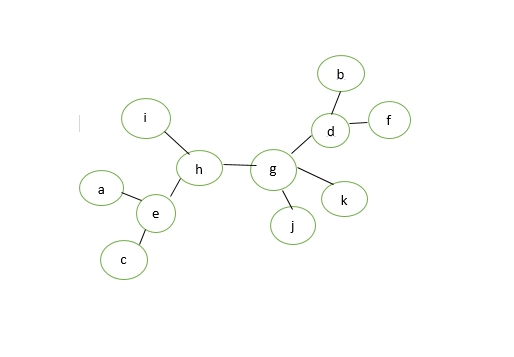• This Graph is not a Tree: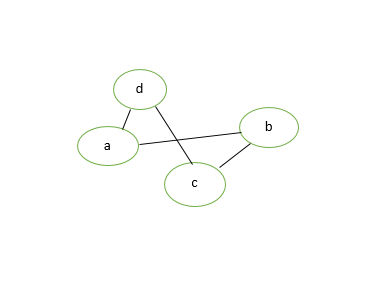Some theorems related to trees are:

• Theorem 1: Prove that for a tree (T), there is one and only one path between every pair of vertices in a tree.

Proof: Since tree (T) is a connected graph, there exist at least one path between every pair of vertices in a tree (T). Now, suppose between two vertices a and b of the tree (T) there exist two paths. The union of these two paths will contain a circuit and tree (T) cannot be a tree. Hence the above statement is proved.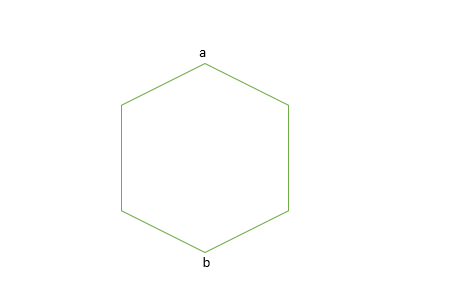Figure 3: Tree(T)

• Theorem 2: If in a graph G there is one and only one path between every pair of vertices than graph G is a tree.

Proof: There is the existence of a path between every pair of vertices so we assume that graph G is connected. A circuit in a graph implies that there is at least one pair of vertices a and b, such that there are two distinct paths between a and b. Since G has one and only one path between every pair of vertices. G cannot have any circuit. Hence graph G is a tree.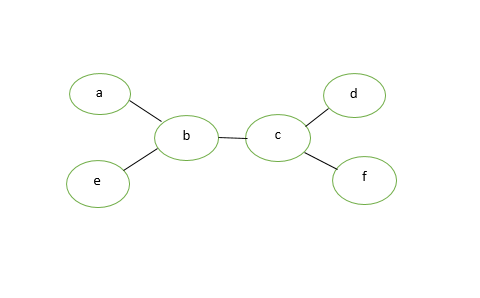Figure 4: Given graph G

• Theorem 3: Prove that a tree with n vertices has (n-1) edges.

Proof: Let n be the number of vertices in a tree (T).
If n=1, then the number of edges=0.
If n=2 then the number of edges=1.
If n=3 then the number of edges=2.

Hence, the statement (or result) is true for n=1, 2, 3.

Let the statement be true for n=m. Now we want to prove that it is true for n=m+1.

Letbe the edge connecting vertices say Vi and Vj. Since G is a tree, then there exists only one path between vertices Vi and Vj. Hence if we delete edge e it will be disconnected graph into two components G1 and G2 say. These components have less than m+1 vertices and there is no circuit and hence each component G1 and G2 have m1 and m2 vertices.

```Now, the total no. of edges = (m1-1) + (m2-1) +1
= (m1+m2)-1
= m+1-1
= m.
```

Hence for n=m+1 vertices there are m edges in a tree (T). By the mathematical induction the graph exactly has n-1 edges.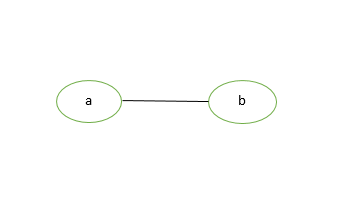Figure 5: Given a tree T

• Theorem 4: Prove that any connected graph G with n vertices and (n-1) edges is a tree.

Proof: We know that the minimum number of edges required to make a graph of n vertices connected is (n-1) edges. We can observe that removal of one edge from the graph G will make it disconnected. Thus a connected graph of n vertices and (n-1) edges cannot have a circuit. Hence a graph G is a tree.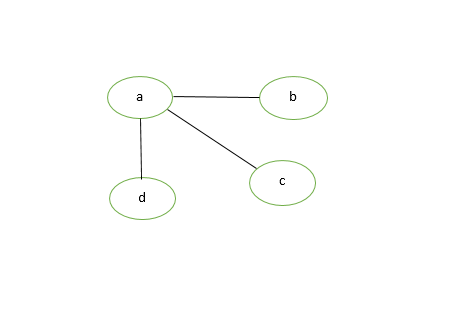Figure 6: Graph G

• Theorem 5: Prove that a graph with n vertices, (n-1) edges and no circuit is a connected graph.

Proof: Let the graph G is disconnected then there exist at least two components G1 and G2 say. Each of the component is circuit-less as G is circuit-less. Now to make a graph G connected we need to add one edge e between the vertices Vi and Vj, where Vi is the vertex of G1 and Vj is the vertex of component G2.
Now the number of edges in G = (n – 1)+1 =n.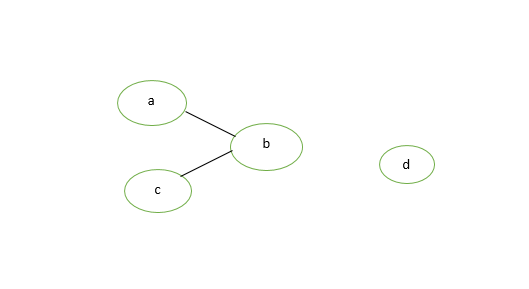Figure 7: Disconnected Graph

Now, G is connected graph and circuit-less with n vertices and n edges, which is impossible because the connected circuit-less graph is a tree and tree with n vertices has (n-1) edges. So the graph G with n vertices, (n-1) edges and without circuit is connected. Hence the given statement is proved.Figure 8:Connected graph G

• Theorem 6: A graph G is a tree if and only if it is minimally connected.

Proof: Let the graph G is minimally connected, i.e; removal of one edge make it disconnected. Therefore, there is no circuit. Hence graph G is a tree.
Conversely, let the graph G is a tree i.e; there exists one and only one path between every pair of vertices and we know that removal of one edge from the path makes the graph disconnected. Hence graph G is minimally connected.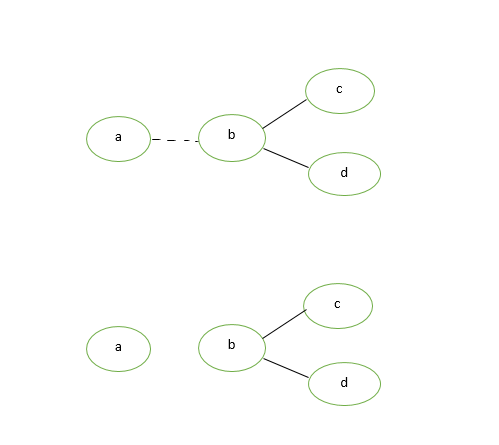Figure 9: Minimally Connected Graph

• Theorem 7: Every tree with at-least two vertices has at-least two pendant vertices.

Proof: Let the number of vertices in a given tree T is n and n>=2. Therefore the number of edges in a tree T=n-1 using above theorems.

```summation of (deg(Vi)) = 2*e
= 2*(n-1)
=2n-2
```

The degree sum is to be divided among n vertices. Since a tree T is a connected graph, it cannot have a vertex of degree zero. Each vertex contributes at-least one to the above sum. Thus there must be at least two vertices of degree 1. Hence every tree with at-least two vertices have at-least two pendant vertices.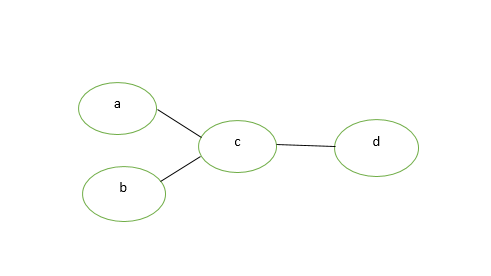Figure 10: Here a, b and d are pendent vertices of the given graph

• Theorem 8: Show that every tree has either one or two centres.

Proof: We will use one observation that the maximum distance max d(v, w) from a given vertex v to any other vertex w occurs only when w is pendant vertex.

Now, let T is a tree with n vertices (n>=2)

T must have at least two pendant vertices. Delete all pendant vertices from T, then resulting graph T’ is still a tree. Again delete pendant vertices from T’ so that resulting T” is still a tree with same centers.

Note that all vertices that T had as centers will still remain centers in T’–>T”–>T”’–>….

continue this process until the remaining tree has either one vertex or one edge. So in the end, if one vertex is there this implies tree T has one center. If one edge is there then tree T has two centers.

• Theorem 9: Prove the maximum number of vertices at level ‘L’ in a binary tree is, where L>=0.

Proof: The given theorem is proved with the help of mathematical induction. At level 0 (L=0), there is only one vertex at level (L=1), there is onlyvertices.

Now we assume that statement is true for the level (L-1).

Therefore, maximum number of vertices on the level (L-1) is. Since we know that each vertex in a binary tree has the maximum of 2 vertices in next level, therefore the number of vertices on the level L is twice that of the level L-1.

Hence at level L, the number of vertices is:-

`*=.`

My Personal Notes arrow_drop_upCheck out this Author's contributed articles.

If you like GeeksforGeeks and would like to contribute, you can also write an article using contribute.geeksforgeeks.org or mail your article to contribute@geeksforgeeks.org. See your article appearing on the GeeksforGeeks main page and help other Geeks.

Please Improve this article if you find anything incorrect by clicking on the "Improve Article" button below.

Improved By : jarretchng02

Article Tags :
Practice Tags :

1

Please write to us at contribute@geeksforgeeks.org to report any issue with the above content.